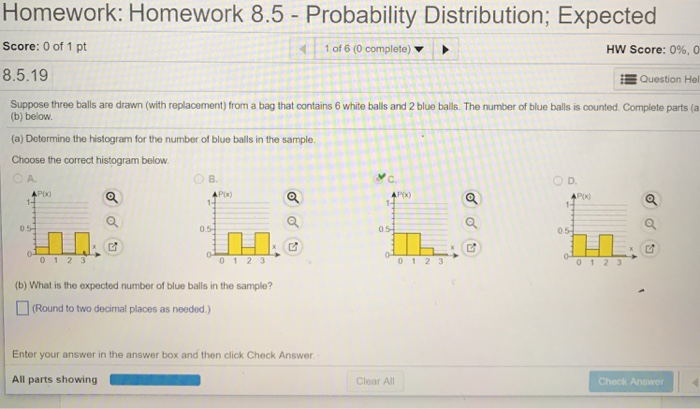# Homework: Homework 8.5 - Probability Distribution; Expected Score: 0 of 1 pt 1 of 6 (0 complete) HW Score: 0%, 0 8.5.19 Question Hel Suppose three balls are drawn (with replacement) from a bag that contains 6 white balls and 2 blue balls. The number of blue balls is counted Complete parts (a (b) below. (a) Dotermine the histogram for the number of blue balls in the sample. Choose the correct histogram below A. O D. Px) P(x) 0.5 0 1 2 3 (b) What is the expected number of blue balls in the sample? (Round to two decimal places as needed ) Enter your answer in the answer box and then click Check Answer Clear All Chack Answer

Questionhelp_outlineImage TranscriptioncloseHomework: Homework 8.5 - Probability Distribution; Expected Score: 0 of 1 pt 1 of 6 (0 complete) HW Score: 0%, 0 8.5.19 Question Hel Suppose three balls are drawn (with replacement) from a bag that contains 6 white balls and 2 blue balls. The number of blue balls is counted Complete parts (a (b) below. (a) Dotermine the histogram for the number of blue balls in the sample. Choose the correct histogram below A. O D. Px) P(x) 0.5 0 1 2 3 (b) What is the expected number of blue balls in the sample? (Round to two decimal places as needed ) Enter your answer in the answer box and then click Check Answer Clear All Chack Answer fullscreen

### Want to see the step-by-step answer?